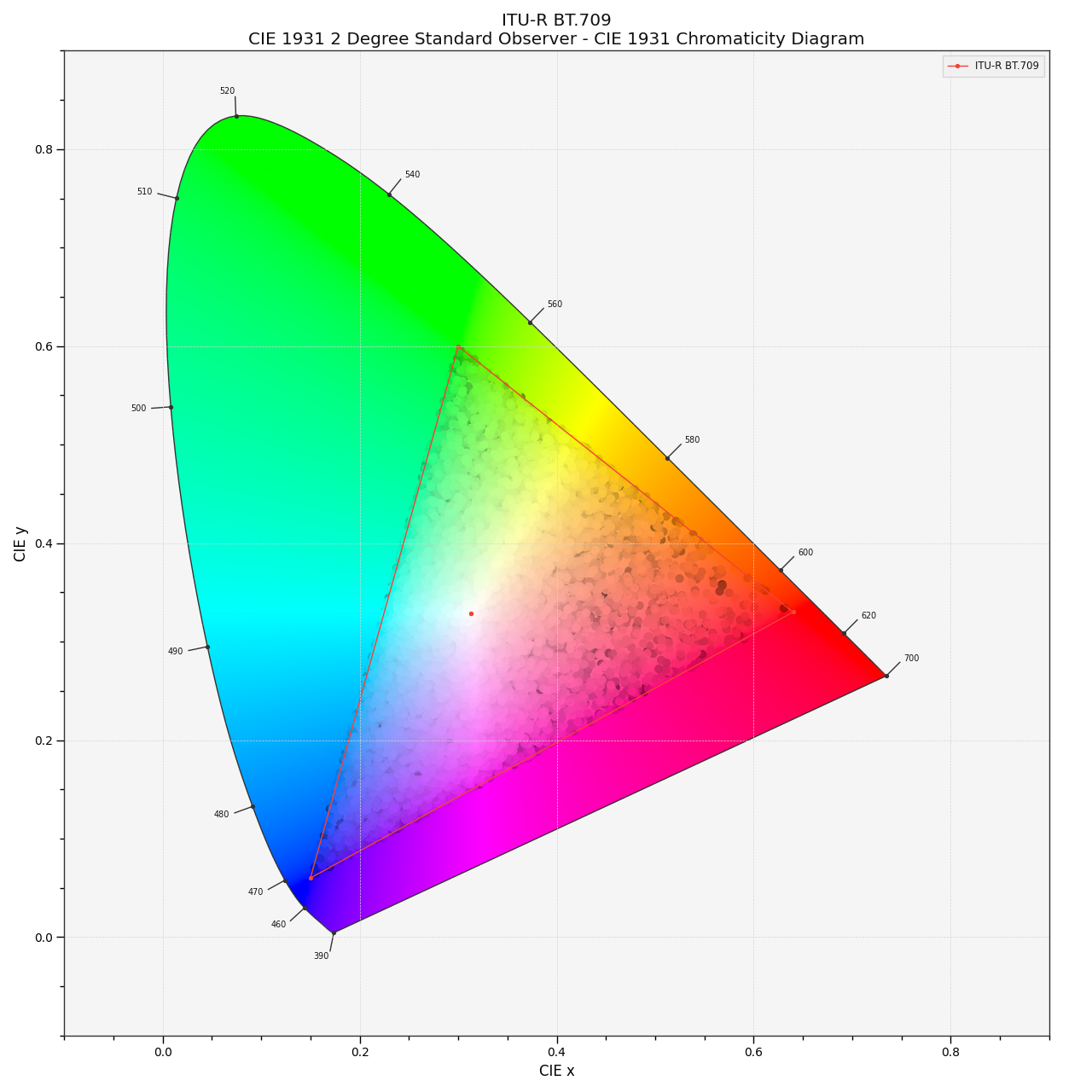# colour.plotting.plot_RGB_chromaticities_in_chromaticity_diagram_CIE1931#

colour.plotting.plot_RGB_chromaticities_in_chromaticity_diagram_CIE1931(RGB: ArrayLike, colourspace: = 'sRGB', chromaticity_diagram_callable_CIE1931: Callable = plot_RGB_colourspaces_in_chromaticity_diagram_CIE1931, scatter_kwargs: = None, **kwargs: Any) [source]#

Plot given RGB colourspace array in the CIE 1931 Chromaticity Diagram.

Parameters:
Returns:

Current figure and axes.

Return type:

`tuple`

Examples

```>>> RGB = np.random.random((128, 128, 3))
>>> plot_RGB_chromaticities_in_chromaticity_diagram_CIE1931(
...     RGB, "ITU-R BT.709"
... )
...
(<Figure size ... with 1 Axes>, <...Axes...>)
```